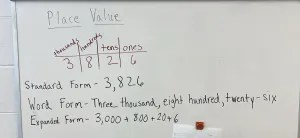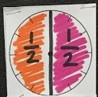# Mathematical Vocabulary- Part 2

In my last vocabulary blog post I discussed the need for teachers and students to use proper mathematical vocabulary. I referenced the 8 Standards of Mathematical Practice and how communication is key in students making sense of problems, reasoning abstractly and quantitatively, constructing viable arguments and critiquing the reasoning of others, and modeling with mathematics.  The 8 Standards of Mathematical Practice leads us to attending to precision as mathematicians. If you have not read that post, I highly encourage you to start there

Today’s blog post is going to focus on the vocabulary teachers and students need to be using when it comes to place value. I am going to try my best to not bring computation strategies into this post, as I will focus on the vocabulary with computation in another post. Stay tuned for that.

As a teacher who taught in the intermediate grades for 15 years, I was constantly trying to tie what we were working on in 4th grade back to what my students knew from kindergarten through 3rd grade. That’s a common idea, make connections to their prior knowledge. One thing I did that made a HUGE difference in my student understanding was how I spoke about numbers. I started using unit form more regularly and I noticed a huge change in how my students were able to manipulate their numbers and build their number sense.

An important thing for students to be able to do is to name numbers differently. If students don’t have a strong number sense, they struggle to be able to see the various ways numbers can be named or decomposed. If we show students the number 170, many of our students see this as one hundred seventy. Few students see this number as seventeen tens. If we start to introduce unit form to our mathematicians, we can help them build a stronger number sense from whole numbers to fractions and decimals.

One of the best ways we can build this number sense is through the use of manipulatives. I’ve had many students struggle with the idea of renaming of numbers and getting out base-ten blocks has been my key to success. We start by having the students show me 1 ten and I ask them what the value of one ten. They look at me like I’m crazy and say, “ten.” I write that out as 10. Then I ask them to show me 2 tens. They have two tens in their hands and I ask again,  “What is the value?” They humor me and say, “Twenty.”  I write out 20. We keep this going until we hit 90. When all of the numbers are written out, like below, I ask them, “What do you notice?”

• 1 ten = 10
• 2 tens = 20
• 3 tens =30
• 4 tens = 40
• 5 tens = 50
• 6 tens = 60
• 7 tens = 70
• 8 tens = 80
• 9 tens = 90

I’m looking for the students to respond with, “Every ten ends in one zero.” When I get that answer out of them, I underline all of the zeros that the tens end in. We then keep going.

• 1 ten = 10
• 2 tens = 20
• 3 tens =30
• 4 tens = 40
• 5 tens = 50
• 6 tens = 60
• 7 tens = 70
• 8 tens = 80
• 9 tens = 90
• 10 tens = 100
• 11 tens = 110
• 12 tens = 120
• 13 tens = 130
• 14 tens = 140

As I write this out for the students to see they really start to notice the pattern. I will then skip ahead and ask them, “What’s 17 tens?” Once they have seen the pattern of every ten ends in 1 zero, they really catch on and can use what they now to know that 17 tens = 170.

We carry this same idea on for hundreds. We start again by having the students show me one hundred block and I ask, “What’s the value of one hundred?” They start to predict where I’m going and reply, “One hundred.”  I write that out as 100. Then I ask them to show me two hundreds. They have two hundreds in their hands and we repeat the process. “What is the value in your hands now?,” I ask. They tell me, “Two hundreds.” I write out 200. We keep this going until we hit 900.

• 1 hundred= 100
• 2 hundreds= 200
• 3 hundreds=300
• 4 hundreds= 400
• 5 hundreds= 500
• 6 hundreds= 600
• 7 hundreds= 700
• 8 hundreds= 800
• 9 hundreds= 900

Again, I ask, “What do we notice about every one of our hundreds?” I’m looking for the students to respond with, “every hundred ends in two zeros.” So we keep going.

• 1 hundred= 100
• 2 hundreds= 200
• 3 hundreds=300
• 4 hundreds= 400
• 5 hundreds= 500
• 6 hundreds= 600
• 7 hundreds= 700
• 8 hundreds= 800
• 9 hundreds= 900
• 10 hundreds= 1000
• 11 hundreds = 1100
• 12 hundreds = 1200
• 13 hundreds = 1300
• 14 hundred = 1400

I will then skip around and ask for the value of 28 hundreds, 42 hundreds, 113 hundreds, and so on. We talk about how we can say 42 hundreds as four thousand two hundred or forty-two hundred. The same number can be seen and said in multiple ways. This is very important when our students get into multi-digit multiplication and division.

We then use what we know about tens and hundreds and make the connection to thousands. As we start to write out the value for various amounts of thousands, the students notice that every thousand ends in 3 zeros. I give them more complicated thousands to write out and they really enjoy using the ideas that they discovered of every ten ends in 1 zero, every hundred ends in 2 zeros, and every thousand ends in 3 zeros. When students discover ideas and concepts, when we give them time to be curious and wonder, we allow them the space and opportunity to develop ownership of that idea.

Using unit form is helpful in other situations as well. For instance, with the number 3,427. One of the standards across grade levels is to write this number in standard form, expanded form, and word form. What’s missing in this is unit form. Instead of only expecting…

3,826 =  three thousand, eight hundred twenty-six = 3000 + 800 + 20 + 6

I actually saw this exact concept  just this week in a 3rd grade intervention classroom I stopped in. There is absolutely nothing wrong with this. I would just like to see the unit form added to the various forms as it will lend to making more sense to students.We can have our students also thinking in terms of units also, which leads us to students combining like units when they get into 6th grade and up. Start with me here.

3,427 + 2,160  =

3 thousands, 4 hundreds, 2 tens, 7 ones + 2 thousands, 1 hundred, 6 tens, 0 ones =

We are going to combine “like units”… thousands with thousands, hundreds with hundreds, tens with tens, and ones with ones. There may come a time where we need to regroup or bundle within our problem, but to start, we need to really focus on the concept of naming our units and combining like units.

3 thousands + 2 thousands = 5 thousands

4 hundreds + 1 hundred = 5 hundreds

2 tens + 6 tens = 8 tens

7 ones + 0 ones= 7 ones

5 thousands 5 hundreds 8 tens 7 ones = 5,587

This allows our students to be using place value vocabulary. As I mentioned, this leads us to some algebraic standards in middle school when students have to start combining like terms or units.

3x + 5y + 7x + 6y =

The x’s and y’s are like our tens or hundreds.

3x + 5y + 7x + 6y

We combine like units.

10x + 11y

This is another computation strategy, partial sums. There are many different ways to show mathematical work for partial sums, but essentially that’s what we are doing, . We are decomposing the problem, or breaking the problem down into parts. We are then putting the parts together to find the whole.

We can do the same thing with decimals. Let’s try 1.578 – 0.346. Here we are still going to write our number in unit form and combine like terms/units… only this time we are subtracting and will be finding partial differences.

1.578- 0.346=

1 whole 5 tenths 7 hundredths 8 thousandths – 0 whole 3 tenths 4 hundredths 6 thousandths

1 whole – 0 whole = 1 whole

5 tenths – 3 tenths = 2 tenths

7 hundredths – 4 hundredths = 3 hundredths

8 thousandths – 6 thousandths = 2 thousandths

1 whole 2 tenths 3 hundredths 2 thousandths = 1.232

My favorite part of naming units comes into play when we are talking fractions. Every year when I am in the second day of school and during our Daily Number Sense Routine working with our “Fraction of the Day”  we are adding 1/2+1/2 . The misconception with students is that 1/2+1/2=1/4. I actually love when a student gives me this answer. I love when students give me wrong answers. It allows me to have a teachable moment. In this instance, I wrote this out on the board.I then ask them, “ What are we counting in this problem?” It’s the second day of school, so they’re all looking around at one another. Sometimes a brand new 4th grader will say that we are counting halves, but not all of the time. I then tie it back to something they did back in kindergarten and I grab a few of those plastic bears all of our primary friends have.

I count them out.1 bear + 1 bear + 1 bear + 1 bear + 1 bear + 1 bear = ?

I’m hoping they say 6 bears. I ask them what we are counting and they say bears. I then refer back to our fraction sentences.

1/2+1/2 = 1 half + 1 half = ?

At this point they usually catch on and tell me 1 half + 1 half = 2 halves. It also helps to have the image of the halves colored in so they can see this circle is not cut into fourths or 4 parts. The circle is cut into halves or 2 parts, with both of them shaded in.I go on to talk about how the number we are saying is the adjective (descriptive word) and the unit is the noun (the thing we are counting). I created these Fraction Vocab cards for fractions to hang in my room and talk about the fraction piece a little further in this blog post on my friend Kristin’s site.

I know I said I wouldn’t get into computation, but when we are naming units, it’s an easy transition into how we are working with students and computation strategies. My next vocabulary post will be more focus on students understanding that as we move to the left on the place value chart we are multiplying by 10 and when we move to the right we are dividing by 10. Stay tuned!